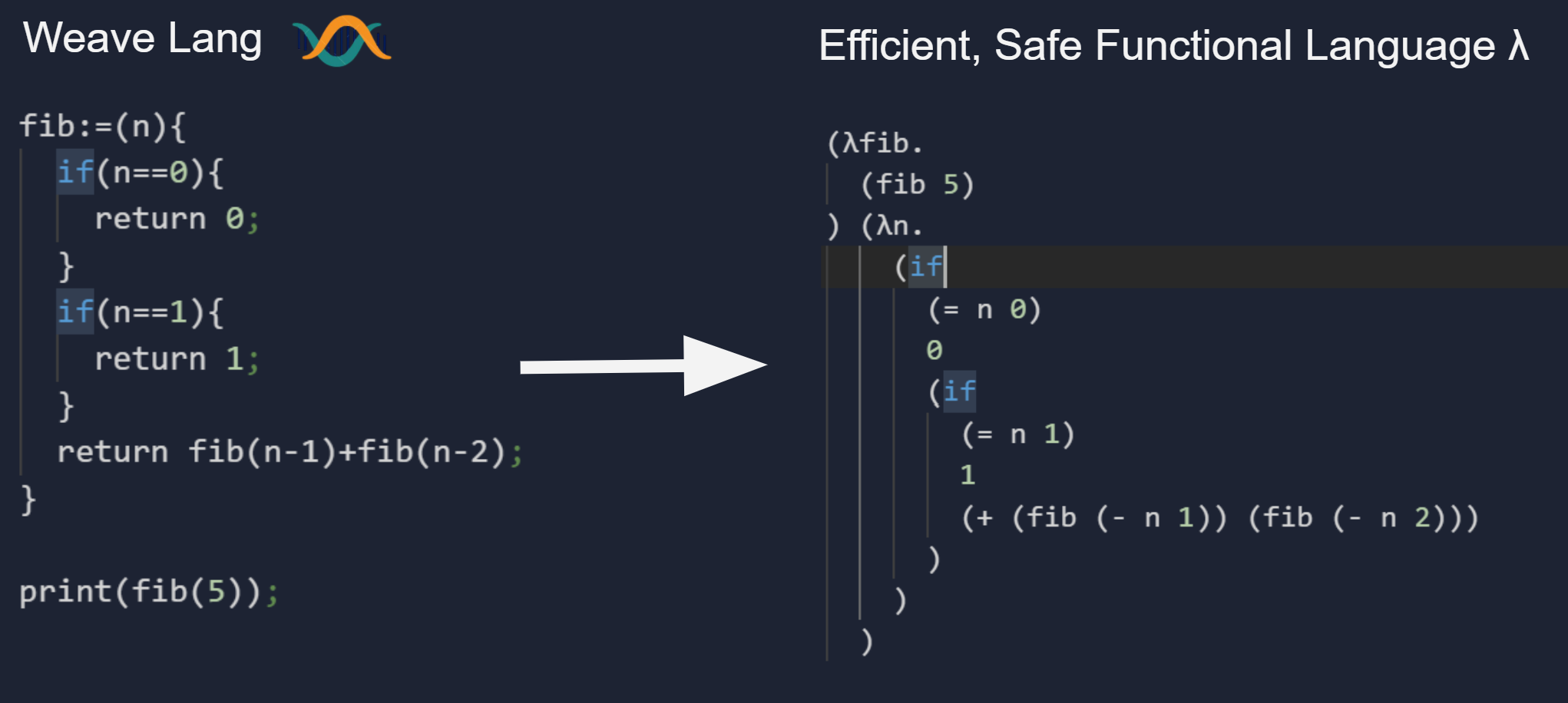Weave Programming Language

# Weave Programming Language

(Created during the Repl.it Programming Language Jam 2020)
Github Repl.itAuthors: Elijah Johnson, Zakery Clarke
Studying Computer Science at the University of New Mexico

Welcome to Weave Programming Language!
Weave is a programming language that transforms imperative style code into efficient functional code. Programming in functional languages is incredibly powerful- offering unmatched expressiveness and program safety. However, programming in a functional style can often be a daunting task.

We have created the Weave programming language to allow C-like programs that take advantage of the flexibility, efficiency and safety of functional languages.

## Features

• Compiles imperative style coding => efficient functional lambda calculus
• Type Inference and strong type checking

### What makes Weave better than C?

• Types can be inferred
• Higher Order Functions
• Strong typing and memory safety

### What makes Weave better than Haskell?

• More comfortable coding style
• Not confined to functional paradigm

## Sample Programs

### Hello World

``print("Hello World!");``

### Factorial

``````factorial:=(n){
if (n <= 1) {
return 1;
}
return n*factorial(n-1);
}

print(factorial(10));``````

### For Loops

``````for(i,[1:10]){
print(i);
x[i]=i;
}
print(x);//Print a single element
print(x);//Print whole array``````

### Fibonacci

``````fib:=(n){
if(n==0){
return 0;
}
if(n==1){
return 1;
}
return fib(n-1)+fib(n-2);
}

print(fib(5));``````

### FizzBuzz

``````for(i,[1:100]){
if (i % 15 == 0) {
print("FizzBuzz");
} else if (i % 3 == 0) {
print("Fizz");
} else if (i % 5 == 0) {
print("Buzz");
} else {
print(i);
}
}``````

We have many ideas to improve Weave before releasing v 1.0! We have outlined many of them below:

• Pattern Matching
``````//Proposed Syntax
fact(1):={return 1;}
fact(n):={return n*fact(n-1);}``````
• Lazy Evaluation
``````//Allows for infinite data structures
naturalNumbers:=pair(1,map((+1),naturalNumbers));
take(10,naturalNumbers) => [1,2,3,4,5,6,7,8,9,10]``````
• Abstract Data types
``````//Create your own data types!
type Suite = Clubs | Diamonds | Hearts | Spades
type Point = Point(Number,Number)
type Shape = Circle(Point,Number) | Rectangle(Point,Point)``````
• Liquid typing
``````factorial(n){
n>=0; //Ensure n is positive
n::=Integer; //Ensure n is integer;
if(n<=1){
return 1;
}
return n*factorial(n-1);
}``````